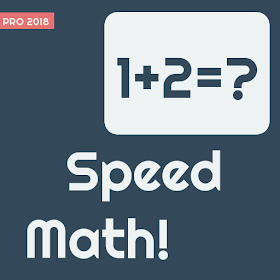# Speed Math 2018 – Ad free Apk v0.0.4 Full Paid

Rate this postSpeed math 2018 is a quick math master game that can make you freaking mad. In this math operations master game we give you very short time (1.5 sec approx) to decide whether the equation that appears on the screen is true or false. This math game is the only game that will give you an option to choose (Plus, Minus, Multiplication and Mix) category by pressing the green button.

Playing Speed Math is a quick exercise for your brain. Speed Math can put your mind into a real challenge.

Game tags:
speed math, math game, kids math games, math games, math mania, math master, freaking math, mathematics, simple math, math practice.

How to play?
* You have only one second to choose the right or wrong.
* You can choose Plus, Minus, Multiplication or Mix type.
* Make the highest score you can.

New Features:
* Plus
* Minus
* Multiplication
* Mix
* Sound on/off

** Do not play if you are not smart enough because this can make you freaking mad**

Note:
Speed Math 2018 is a clone of “freaking math” with some additional features.

## Screenshots Of Speed Math 2018 – Ad free Apk v0.0.4 Full Paid

App Requirements:

Android – 4.1+

Version – 0.0.4

Size – 4.9 MB